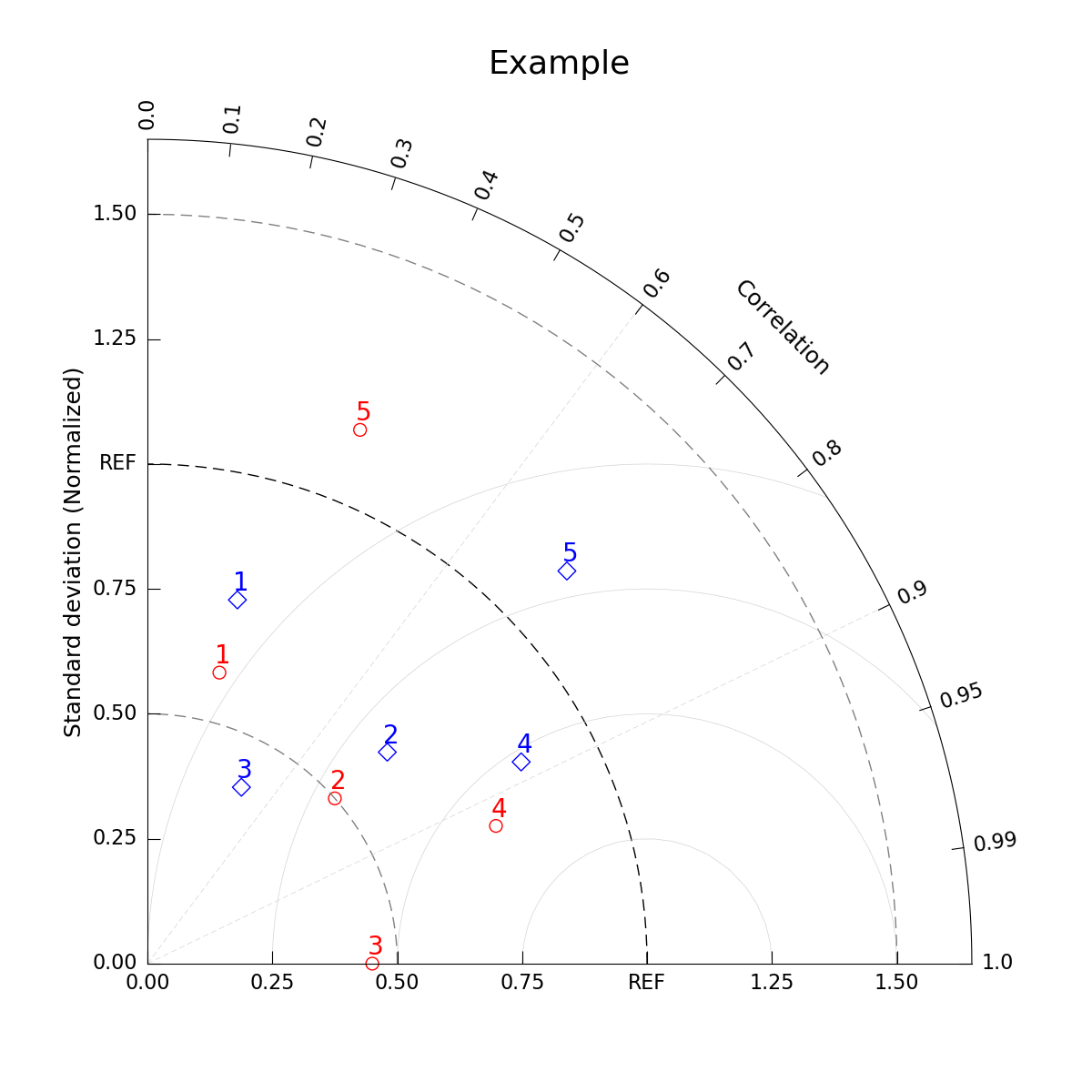# NCL_taylor_2.py#

Concepts illustrated:
• Creating a simple Taylor Diagram

• Adding background options for the diagram

See following URLs to see the reproduced NCL plot & script:
Note: Due to to limitations of matplotlib’s axisartist toolkit, we cannot include minor tick marks

between 0.9 and 0.99, as seen in the original NCL plot.

Import packages:

```import matplotlib.pyplot as plt
import numpy as np

import geocat.viz as gv
```

Create dummy data:

```# p dataset
pstddev = [0.6, 0.5, 0.45, 0.75, 1.15]  # standard deviation
pcorrcoef = [0.24, 0.75, 1, 0.93, 0.37]  # correlation coefficient

# t dataset
tstddev = [0.75, 0.64, 0.4, 0.85, 1.15]
tcorrcoef = [0.24, 0.75, 0.47, 0.88, 0.73]
```

Plot

```# Create figure and Taylor Diagram instance
fig = plt.figure(figsize=(12, 12))
dia = gv.TaylorDiagram(fig=fig, label='REF')
ax = plt.gca()

# Add model sets for p and t datasets
pstddev,
pcorrcoef,
fontsize=20,
xytext=(-5, 10),  # marker label location, in pixels
color='red',
marker='o',
facecolors='none',
s=100)  # marker size
tstddev,
tcorrcoef,
fontsize=20,
xytext=(-5, 10),  # marker label location, in pixels
color='blue',
marker='D',
facecolors='none',
s=100)

# Add RMS contours, and label them
colors='lightgrey',
linewidths=0.5)